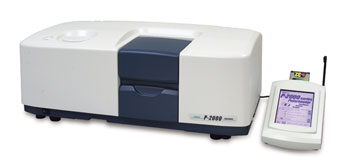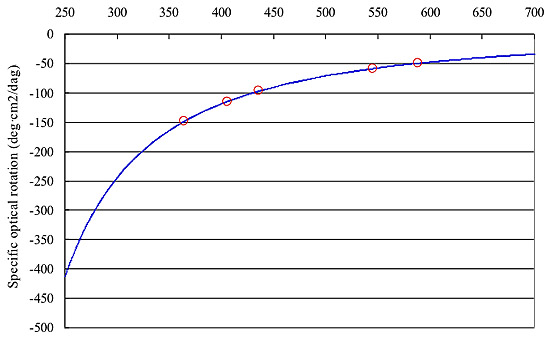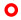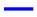# Polarimetry and Optical Rotation of Sucrose and l-menthol

May 28, 2017

## Polarimetry and Measurement of Sucrose and Menthol

Polarimetry is the measurement of the optical rotation of a substance that rotates the plane of polarization of linearly-polarized light as it passes through it. This is more specifically defined as the property of an optically active substance where the refractive indices for left and right circularly-polarized light are different. Optical rotation in a clockwise direction (as viewed towards the light source) is defined as dextrorotation (+) and the opposite, levorotation (-).

The angle of rotated plane of polarization is called optical rotation and a polarimeter is used instrument to measure the degree of optical rotation. Optical rotation is proportional to both cell path length and sample concentration, and dependent on wavelength and temperature.

Specific optical rotation [α]tx is calculated using the following formula with the terms: temperature t (°C), wavelength x (nm), cell pathlength l (dm), sample concentration c (g/100 ml) and measured optical rotation α.

[α]tx = 100 α/cl

JP, USP and EP require measurement of optical rotation using the Sodium D emission line . Polarimetry is used for several purposes including purity certification of sugar, verification of pharmaceuticals and optical purity determination of optical active substances obtained from asymmetric synthesis in organic chemistry.

### Optical Rotation Measurement of SucroseP-2000 Digital Polarimeter

5 g/100ml of sucrose (Wako Pure Chemical Industries, Ltd., JIS special grade) was prepared and measured using a P-2000 with 100mm cell, Na lamp D589 at 20°C.

Figure 1 Printout view of the results. The average of optical rotation measured 5 times was +3.3264 deg. and its calculated specific optical rotation was +66.5280 which shows excellent correlation with the specific optical rotation of 5g/100ml sucrose solution described in JIS K0063 1) , +66.500Figure 1. Printout view of optical rotation measurement of 5g/100ml sucrose solution.

### Optical Rotation Measurement of 1-menthol

l-menthol (Wako Pure Chemical Industries, Ltd.) was prepared as per JP (2.5g ethanol (95), 25ml, 100mm) and the optical rotation was measured. The specific optical rotation was calculated as [α]20D = -50.01 which is well within the JP criteria: -45.0 ~ -51.0.

Generally, optical rotation increased at shorter wavelengths. For optical rotation measurement,  the Na lamp D589 is normally used, however when the optical rotation of a sample is small using the D-line,  shorter wavelengths may provide a better result. In this application data the following were compared, optical rotation measured with both Na589 and a Hg lamp with emission line wavelengths (546, 436, 405, 365 nm) and a complete ORD spectrum measured using a J-820 spectropolarimeter with ORD attachment. As shown in Figure 2, the measured values by both P-2000 and ORDM-401 show strong correlation. By employing shorter wavelengths for samples with small optical rotation, the measurement can be improved significantly.Figure 2. Optical rotation of 1-menthol and comparison with ORD spectrumP-2000 measured value: with ORD spectrum

### About the Author

Leah Pandiscia received her PhD from Drexel University where she studied Biophysical Chemistry. She is a Spectroscopy Applications Scientist.